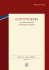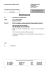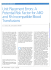# A MINIMAX DISTRIBUTION FREE PROCEDURE FOR

## Transcription

A MINIMAX DISTRIBUTION FREE PROCEDURE FOR
```Journal of the Operations Research
Society of Japan
Vol. 42, No. 3, September 1999
© 1999 The Operations Research Society of Japan
A MINIMAX DISTRIBUTION FREE PROCEDURE FOR STOCHASTIC
INVENTORY MODELS WITH A RANDOM BACKORDER RATE
Liang-Yuh Ouyang
Bor-Ren Chuang
Tamkang University
Abstract
The stochastic inventory models analyzed in this paper involve two models that are continuous
review and periodic review in which the backorder rate is a random variable. For these two models with
a mixture of backorders and lost sales, we respectively assume that their mean and variance of lead time
demand and review period demand are known, but their probability distribution are unknown. Instead of
having a stockout cost term in the objective function, a service level constraint is added to the models.
We develop a procedure t o find the optimal solution for each case. Furthermore, the sensitivity analysis is
performed.
1. Introduction
Among the modern production management, the Japanese successful experiences of using
Just-In-Time (JIT) production show that the advantages and benefits associated with efforts
to control the lead time can be clearly perceived. The goal of JIT inventory management
philosophies is the focus which emphasizes high quality, keeps low inventory level and lead
time to a practical minimum. In 1983, Monden [l]studied Toyota production system, and
clearly declared that shortening lead time is a crux of elevating productivity.
In most of the early literature dealing with inventory problems, either in deterministic
or probabilistic model, lead time is viewed as a prescribed constant or a stochastic variable,
which therefore, is not subject to control (see, e.g., Naddor  and Silver and Peterson ).
Recently, there have been some inventory literature which consider lead time as a decision
variable. Liao and Shyu  first presented a continuous review inventory model in which the
order quantity was predetermined and lead time was a decision unique variable. Ben-Daya
and Raouf [S] extended Liao and Shyu's  model by considering both the lead time and
order quantity to be decision variables where shortages were neglected. Ouyang et al. 
generalized Ben-Daya and Raouf's  model by allowing shortages and the total amount
of stockout is considered as a mixture of backorders and lost sales. In a recent research
article, Ouyang and Wu  considered an inventory model with a mixture of backorders
and lost sales in which a service level constraint was used instead of shortage cost in the
objective function. However, in those models previously mentioned [5-71, reorder point had
not been taken into account, and merely focused on the relationship between lead time and
order quantity; that is, they neglected the possible impact of reorder point on the economic
ordering strategy. In this article, we attempt to allow the reorder point as one of the decision
variables in the modeling.
In addition, for practical inventory system, shortages are unavoidable due to various
uncertainties. While a demand is unsatisfied during the lead time, generally there exists
a mixture of backorders and lost sales; but existing literature mainly discussed that the
A Minimax Distribution Free Inventory Models
backorder rate was a fixed constant. In this article, we consider that customers' patience is
hard to estimate, and hence we here allow the backorder rate to be a random variable to
agree with the real inventory environment.
In this study, we adopt Liao and Shyu's  assumption, which suppose that lead time can
be decomposed into n mutually independent components each having a different crashing
cost for reducing lead time. We also assume that instead of having a stockout cost term
in the objective function, a service level constraint is added to the models. Two purposes
of this paper are to establish a (Q, r, L) inventory model for the continuous review case
and to propose a new (T, R, L) inventory model for the periodic review case. For these
two models with a mixture of backorders and lost sales, we respectively consider that the
form of the probability distribution of lead time demand and review period demand is
unknown, and merely assume that their first and second moments are known (and hence,
mean and variance are also known), and solve these inventory models by using the minimax
distribution free approach. Moreover, the sensitivity analysis is included and two illustrative
numerical examples are provided.
2. Notations and Assumptions
The mathematical models in this paper are developed on the basis of the following notations
and assumptions.
Notations :
D
= expected demand per year
A
= ordering cost per order
h
= holding cost per unit per year
= mean of the demand per unit time
P
0
= variance of the demand per unit time
a
= proportion of demands that are not met from stock. Hence, 1 - a is the
service level, 0 < a < 112
=
the fraction of the demand during the stockout period that will be
j3
backordered, 0 <: Q 1, a random variable
g(j3) = the probability density function (p.d.f .) of 9
Q
= order quantity, a decision variable
= reorder point for the continuous review case, a decision variable
r
R
= target inventory level for the periodic review case, a decision variable
L
= length of lead time, a decision variable
T
= length of a review period, a decision variable
X
= the lead time (or revrew period plus lead time) demand which has
a p-d.f. f x ( X )
E ( - ) = mathematical expectation
X+
= maximum value of X and 0, i.e., X^ = Max{x, O}.
<
Assumptions :
1. The lead time L consists of n mutually independent components. The i-th component
has a minimum duration a; and normal duration b;, and a crashing cost per unit time
c2
cn. Then, it is
c;. Further, for convenience, we rearrange c; such that cl
clear that the reduction of lead time should be first on component 1 because it has the
minimum unit crashing cost, and then component 2, and so on.
2. For the continuous review case, the length of the lead time L does not exceed an inventory
cycle time Q / D so that there is never more than a single order outstanding in any cycle.
< <
- -â
<
L. - Y.Ouyang & B. -R. Chuang
For the periodic review case, the lead time L does not exceed the review period T so
that no more than a single order will be outstanding at any moment.
If the demand per unit time, Y, has a probability distribution with mean pi and variance
c?, then the distribution of the demand during the length of time t is a t-fold; that is,
the demand Y during t follows a p.d.f. fy(y) with mean fit and variance 6%.
Continuous Review Model
this section, we assume that inventory is continuously reviewed. An order quantity of size
Q is ordered whenever the inventory level drops to the reorder point r . The reorder point r
= expected demand during lead time
safety stock, and the safety stock = k x (standard
deviation of the demand during L), i.e., r = p L + k a a , where k is the safety factor. When
the lead time demand X has a p.d.f. fx(x) with mean p L and standard deviation ofi, the
+
/
.
P 0 0
expected demand shortage at the end of cycle is given by E(X - r)+ =
( X - r ) fx(x)dx;
r
and thus the expected number of backorders per cycle is QE(X - r ) + and the expected
number of lost sales per cycle is (1 - ft)E(X - r ) + , where 0 <, ft 1.
he expected net inventory level just before the order arrives is r -fi L (l-/?)Â£( -r)+,
and the expected net inventory level at the beginning of the cycle is Q r - p L (1 ft)E(X- r)+ when an order lot Q arrives. Therefore, the expected holding cost per cycle
<
+ r - f i +~(1 - Q)E(X
+
+
+
- r)+].
Under the Assumption 1 as in the above section, if we let L. denote the length of
lead time with each component having a normal duration, and Li be the length of lead
time with first i components 1, 2, - - - ,1 crashed to their minimum duration and the rest
n
bj and
components are normal duration, then L. and Li can be expressed as L. =
j=l
i
oj
Li =
j= l
+
n
X bj,
i = 1,2, -
j=i+1
. , n, respectively; and hence the lead time crashing cost
i- 1
C(L) per cycle for L E [Li7Li_l] can be written as C(Â£=
- L)
+ X cj(bj - a,).
j= 1
With the preceding statements, the expected total cost per cycle is given by
T (Q, r, L) = ordering cost
+ holding cost + lead time crashing cost
Notice that the stockout cost term is not included in the objective function.
Because the backorder rate Q is a random variable with p.d.f. g(.P\ the expected back-
1
1
order rate is MP =
ftg(Q)dp. And the expected order number per year is D / Q , therefore,
the expected total annual cost is
Our objective is to minimize the expected total annual inventory cost, subject to a
constraint on service level. That is, the mathematical model of this problem can be expressed
A Minimax Distribution Free Inventory Models
as follows:
Subject to
E ( X - r)+
Q
a.
Since the form of the probability distribution of lead time demand X is unknown, we can
not find the exact value of E ( X - r)+. Hence, we use minimax distribution free procedure to
solve our problem. If we let T denote the class of p.d.f.'s with finite mean p L and standard
then the minimax distribution free approach for this problem is to find the
deviation (TV^,
most unfavorable p. d.f. fx in T for each (Q, r , L) and then minimize over (Q, r, L); that is,
our problem is to solve
Subject to
E ( X - r)+
Q
5 a.
For this purpose, we need the following proposition which was asserted by Gallego and
Moon [B].
Proposition
For any fx 6 T,
Moreover, the upper bound (5) is tight.
Given that r = p L + ko'^/L, and for any probability distribution of the lead time demand
X, the above inequality always holds. Then, using inequality (5) and considering the safety
factor k as a decision variable instead of r , the model (4) is reduced to
Min EAC(Q, k, L) = DIA+c(L)l + 9 ' + h 0 f i , k
Q
2
Subject to
(d- k) 5 2aQ.
2
- M&/--^
(6)
aVI
The inequality constraint in model (6) can be converted into equality by adding a nonnegative slack variable, S2.Thus, the Lagrangean function is given by
D[A
+
Q
+ I.Q
+ h ( T A + ;(l
2
^
- Mp) (G-
where A is a Lagrange multiplier.
Notice that, for any given (Q, k, A, S ) , EAC(Q, k, L, A, S ) is a concave function in L 6
[Li, L;because
L.- Y. Ouyang & B. -R. Chuang
Hence, for fixed (Q, k, A, S ) , the minimum expected total annual cost will occur at the end
points of the interval [Li,
On the other hand, we can further prove that, for any
given L G [Li,L;_l], EAC(Q, k9L, A, S) satisfies the Kuhn-Tucker necessary conditions for
minimization problem and it gets the slack variable S2= 0 (hence, the inequality constraint
in model (6) will become an equality). Therefore, for fixed L G [Li,
the minimum
value of function (7) (in which the variable S = 0) will occur at the point (Q9kJ) which
satisfies
and
Solving (8), (9) and (10) for Q, A, and k, respectively, leads to
and
Combining equations (1l ) , (12), and (13), we get the order quantity
Thus, we can establish the following algorithm to find the optimal (Q, k, L).
Algorithm 1
Step 1. For each L,, i = 0,1,2, - - - ,n, we use equation (14) to evaluate Q,, and then use
equation (13) to determine ki.
Step 2. For each (Qi, ki, L,), compute the corresponding expected total annual cost EAC(Qi7
ki.Li)^ i = 0 , 1 , 2 , - - - , n .
Step 3. Find Min EAC(Qi, ki, L;). If EAC(Q*,k*, L*) = Min EAC(Qi, ki, L.), then
i=O,l,2,--,n
1=0,1,2,~,n
(Q*, /c*, L*) is the optimal solution. And hence, the optimal reorder point r* is
r* = pL* + k ' a o .
,
Periodic Review Model
In this section, we assume that the inventory level is reviewed every T units of time and a
sufficient ordering quantity is ordered up to the target inventory level R, and the ordering
quantity is arrived after L units of time. The target inventory level R = expected demand
during the period of length ( T + L) + safety stock, and the safety stock = Sx (standard
deviation of the demand during T + L), i.e., R = p ( T + L) + S a ^ / T m ,where S is a safety
factor. When the demand X during ( T + L) has a p.d.f. fx (X) with mean p ( T + L) and
4.
A Minimax Distribution Free Inventory Models
standard deviation o \ / T + , the expected demand shortage at the end of cycle is given
by E ( X - R)' = I w ( x - R) fx(x)dx, and the expected number of lost sales per cycle is
R
(l - f3)E(X - R)+.
By a similar discussion as in Montgomery e t al. [g] to discuss the periodic review case, the
review period T will be used as the time between the arrival of two successive orders rather
than between the placement of two successive orders. Hence, the expected net inventory
level at the beginning of the period is R - p L + (1 - ft)E ( X - R)+, and the expected net
inventory level at the end of the period is R - p(T L) (1 - ft)E(X - R)+. Thus, the
[
+ +
+ (1 ,B)E ( X - R)'].
expected holding cost per year is h R - pL 2
mathematical model of the period review case can be formulated as
Subject to
E ( X - R)'
D{T L)
+
Therefore, the
a.
Based upon the same proposition as presented in the previous continuous review case
and R = p ( T L) S a ^ / T m , we can obtain the following inequality:
+ +
E ( X - R)' 5
\$^/^TT
(m
- 6) ,
+
for any fx 6 T (which is the class of p.d.f, fx7s with finite mean p ( T L) and standard
deviation o \ / T ) . And the upper bound (16) is tight.
On applying this result and allowing the safety factor S as a decision variable instead of
R, the model (15) is reduced to minimize
Subject to
( d m- S) <. 2 a D ( T + L).
Hence, the Lagrangean function can be formulated as
where A is a Lagrange multiplier and S2is a nonnegative slack variable.
By analogous arguments in the continuous review case, it can be verified that EAC(T, S,
L, A, S) is a concave function of L E [LÃ
for fixed (T, S, A, S ) . Thus, for fixed (T, S, A, S),
the minimum value of EAC(T, S, L, A, S) will occur at the end points of the interval [Li, L;-i].
Furthermore, for fixed L E [Li,
Li-11, we can show that EAC(T, S, L, A, S) satisfies the KuhnTucker necessary conditions for minimization problem and it obtains the slack variable
S2 = 0. Consequently, for fixed L E [L;,
the minimum value of function (18) (in
which the variable S = 0) will occur at the point (T,S, A) which satisfies
L. - Y. Ouyang & B. -R. Chuang
and
9EAC(T,S9L, A)
- 0.
QA
Solving equations (19)) (20), and (21) for T, A, and 6. respectively, results in
and
By substituting (23) and (24) into (22)) the review period
The following algorithm can be utilized to find the optimal (T,S, L).
Algorithm 2
Step 1. For each L^ i = 0 , 1 , 2 , - - - , n ,we use equation (25) to evaluate T,, and then use
equation (24) to compute &.
Step 2. For each (T;, &, L;), compute the corresponding expected total annual cost EAC(Ti,
Si, Li), i = 0, l, 2, -
Step^. Find
, n.
Min EAC(Ti74, L,}. If EAC(T*,8*, L*) =
Min E A C ( r , bi, L,), then
(T*,&, L*) is the optimal solution. And hence, the optimal target inventory level
R. is R* = p(T* L*) s * o - / ! m .
1=0,1,2,Â¥-,
,
z=O,l,2,..-,n
+ +
5. Sensitivity Analysis
1. For the continuous review case, observe that the effect of expected backorder rate MD
on the expected total annual cost may be examined. From (6), for fixed Q, k, and L, we
have
This implies when increasing the expected backorder rate, the expected total annual cost
will decrease. The periodic review case has the same property.
2. In the continuous review case, from equation (14), we take the derivatives of Q with
respected to Mp, and obtain
dQ
dM,
-
1 -2*
> 0 (because
a
< 112).
This means that increasing the expected backorder rate MP increase the order quantity
Q-
3. For fixed L E [Lh
from equation (13)) we get
A Minimax Distribution Free Inventory Models
or equivalently,
Hence, for the continuous review model, increasing the service level l - a will result in
an increase in the safety factor k (or, equivalently increases the reorder point r , because
r = pL k a a ) . On the other hand, the periodic review model has the same property,
i.e., increasing the service level I - a will result in an increase in the target inventory
level R.
4. Taking the derivatives of (25) with respect to a, we obtain
+
or equivalently,
It implies that increasing the service level l - a decreases the review period T .
5. Clearly, for the continuous review case, if k is sufficiently large, we get &-+T
-k
then the model (6) is reduced to the model of Ben-Daya and Raouf .
-+ 0,
Numerical E x a m p l e s
Case 1. Continuous review model
In order to illustrate the above solution procedure, let us consider an inventory system
with the data used in Ouyang and Wu : D = 600 units/year, A = \$200 per order, h = \$20
/unit/year, p = 11 units/week, a = 7 unitslweek, the service level 1 - a = 0.985, i.e., the
proportion of demands that are not met from stock is a = 0.015. The lead time has three
components with data as shown in Table 1. Moreover, suppose that the backorder rate ,B
during the stockout period has a uniform distribution, i.e., the p.d.f. of /? is g{P} = 1, 0 5
f3 1; = 0, otherwise. Hence, the mean of /? is Mg = 0.5.
6.
<
component
i
1
Normal Minimum Unit crashing
duration duration
cost
b; (days) a; (days)
c; (\$ /day)
20
6
0.4
For this case, applying the Algorithm 1 procedure yields the results as shown in Table 2.
From Table 2, the optimal inventory policy with a service level constraint can be easily found
by comparing EAC(Qi7R;,L;), 1 = 0,1,2,3, under the stockout level bound a = 0.015.
Therefore, we obtain the optimal order quantity Q* = 142 units, the optimal reorder point
r* = 65 units, and the optimal lead time L* = 4 weeks. The minimum expected total annual
cost EAC(Q*, r*, L*) = \$2,798.23.
R e m a r k : In Ouyang and Wu's  model, which considered a fixed reorder point r (i.e.,
they let P(X > r) = 0.2)) and took {3 = 1 and a = 0.015. They obtained the optimal
(Q*,L*) = (116,4) and the minimum expected total annual cost EAC(116,4) = \$2,839.06.
L. - Y. Ouyang & B.-R. Chuang
Table 2 : Solution procedure of Algorithm 1 (Li in week)
?
1 and a = 0.015 but r to be considered as
In our model, for the same case, i.e., ,l=
a decision variable, we reevaluate and obtain the optimal (Q*,r*, L*) = (124,54,4) and
EAC(124,54,4) = \$2,524.05. Thus, we find that our model savings EAC(Q*, L*) EAC(Q*,r*,L*) = EAC(116,4) - EAC(125,54,4) = \$2,546.94 - \$2,524.05 = \$22.89,
which can be viewed as the rewards due to controlling the reorder point to be a decision
variable.
Case 2. Periodic review model
The data is as in Case 1. Applying the Algorithm 2, we tabulate the results in Table 3.
From Table 3, under the stockout level bound a = 0.015, the optimal value (T*,R*, L*) =
(9.8,263,8), and the minimum expected total annual cost EAC(T., R,, L.) = \$3,522.67.
Table 3 : Solution procedure of Algorithm 2 (Ti, Liin week)
Comparing the optimal results in Table 2 and Table 3, we observe that the periodic
review model is more expensive than the continuous review model and the cost difference
EAC(T*, R*,L*) - EAC(Q*, r\L*) = \$3,522.67 - \$2,798.23 = \$724.44.
7. Concluding Remarks
In this study, we present two inventory models with a mixture of backorders and lost sales,
where the backorder rate be a random variable and the stockout cost term in the objective
function is replaced by a service level constraint. One is the (Q, r, L) inventory model for
the continuous review case and the other is (T, R, L) inventory model for the periodic review
case. For these two models, we respectively consider that the distributional form of lead time
demand and review period demand is unknown, and apply the rninimax decision criterion
to solve the distribution free model.
In future research on this problem, it would be interesting to deal with an arrival order
lot involving some defective it ems.
Acknowledgments
The authors are thankful to the anonymous referees for their constructive suggestions
to improve the paper.
References
[l] Y. Monden: To yota Production System (Institute of Industrial Engineers, Norcross,
A Minimax Distribution Free Inventory Models
Georgia, 1983).
 E. Naddor: Inventory System (John Wiley, New York, 1966).
 E. A. Silver and R. Peterson: Decision Systems for Inventory Management and Production Planning (John Wiley, New York, 1985).
 C. J. Liao and C. H. Shyu: An analytical determination of lead time with normal
demand. International Journal of Operations & Production Management, 11 (1991) 7278.
 M. Ben-Daya and A. Raouf: Inventory models involving lead time as decision variable.
Journal of the Operational Research Society, 4 5 (1994) 579-582.
 L. Y. Ouyang, N. C. Yeh and K. S. Wu: Mixture inventory model with backorders and
lost sales for variable lead time. Journal of the Operational Research Society, 47(1996)
829-832.
 L. Y. Ouyang and K. S. Wu: A rninimax distribution free procedure for mixture inventory
model involving variable lead time. Computers and Operations Research, 24(1997) 875882.
 G. Gallego and I. Moon: The distribution free newsboy problem : Review and extensions.
Journal of the Operational Research Society, 44 (1993) 825-834.
[g] D. C. Montgomery, M. S. Bazaraa and A. I . Keswani: Inventory models with a mixture
of backorders and lost sales. Naval Research Logistics, 20 (1973) 255-263.
Liang-Yuh Ouyang
Department of Management Sciences
Tamkang University
Tamsui, Taipei, Taiwan 25137, R.O.C.
Email: l [email protected] .t ku .edu .t w
```

### Overview The Benefits of AmbuTRAK Bound Tree Medical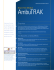### How The Vitamin Shoppe Answered the Inventory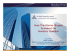### Company Presentation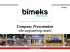### Assessment to Build a Low-Risk, Productive Workforce.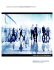### Maytech SDS Users - ECi Software Solutions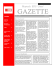### Craigslist Connect 20160107 Tucker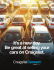### Ticketmaster Archtics® SPORTS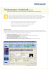### Sage50 Accounting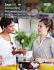### Final MF Project Kate Walker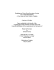### June 18, 2015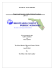### Epicor Manufacturing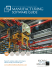### D3.3 Mid term revision of the data management plan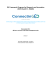### The year in pictures - East African Community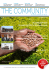### Handbook telc English A2-B1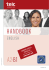### Fall 2008 - Eastern Arizona College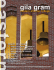### eStore Database/Application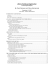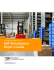### Read the Thruway Fasteners Case Study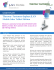### CERS Busin ess Us ser Gu uide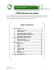### glottotheory - Universität Wien